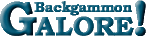Turner's FormulaTurner's Formula A simple formula devised by Stephen Turner for estimating the match equity (1) at a given score. Expressed as a percent, the leader's match equity E = 50 + (24/T + 3) * D, where T is the number of points (4) the trailer still needs and D is the difference in scores. See post by Stephen Turner.  Compare: Janowski's Formula and Neil's Numbers.

 Help   •   About the Glossary   •   List of Terms   •   Full Glossary